## ML Aggarwal Class 6 Solutions for ICSE Maths Chapter 4 Playing with Numbers Ex 4.4

Question 1.
Find the H.C.F. of the given numbers by prime factorisation method :
(i) 28, 36
(ii) 54, 72, 90
(iii) 105, 140, 175.
Solution:
(i) Prime factorisation of the given numbers are :
28 = 2 × 2 × 7
36 = 2 × 2 × 3 × 3
Notice that 2 occurs as a common prime factor atleast 2 times in both given numbers.
∴ H.C.F = 2 × 2 = 4

(ii) Prime factorisation of the given numbers are :
54 = 2 × 3 × 3 × 3
72 = 2 × 2 × 2 × 3 × 3
90 = 2 × 3 × 3 × 5
Notice that 2 occurs as a common prime factor atleast one times and 3 atleast two times in given numbers.
∴ H.C.F = 2 × 3 × 3 = 18

(iii) Prime factorisation of given numbers are :
105 = 3 × 5 × 7
140 = 2 × 2 × 5 × 7
175 = 5 × 5 × 7
Notice that 5 occurs as a common prime factor atleast one time and 7 one time in given numbers.
∴ H.C.F = 5 × 7 = 35

Question 2.
Find the H.C.F. of the given numbers by division method:
(ii) 198, 429
(ii) 20, 64, 104
(iii) 120, 144, 204
Solution:Last remainder = 0, stop here
∴ H.C.F = 33 (Last divisor)

(ii) Let us find H.C.F of 20 and 64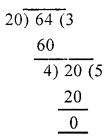∴ H.C.F of 20 and 64 = 4
Now, find H.C.F of 4 and 104∴ H.C.F of 20, 64, 104 = 4

(iii) 120, 144, 204
First, find the H.C.F. of 120 and 144H.C.F. of 120 and 204 is 12
Now, find the H.C.F. of 12 and 144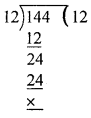∴ H.C.F of 120, 144 and 204 = 12.

Question 3.
Fill in the blanks:
(i) HCF of two consecutive natural numbers is ……
(ii) HCF of two consecutive odd numbers is …..
(iii) HCF of two consecutive even numbers is ……
Solution:
(i) HCF of two consecutive natural numbers is 1.
(ii) HCF of two consecutive odd numbers is 1.
(iii) HCF of two consecutive even numbers is 2.

Question 4.
Find the greatest number which can divide 257 and 329 so as to leave a remainder 5 in each case. .
Solution:
When 257 is divided by the required number, 5 is left as a remainder.
So 257 – 5 = 252 i.e. 252 is exactly divisible by that number.
Similarly 329 – 5 = 324 is exactly divisible by that number.
∴ 252 and 324 are both divisible by that number. Thus, the required number is the H.C.F. of 252 and 324.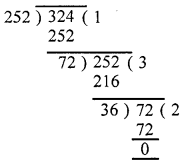Hence the required number = 36

Question 5.
Find the largest number that will divide 623, 729 and 841 leaving remainder 3, 9 and 1 respectively.
Solution:
Numbers are 623, 729 and 841 and remainders are 3, 9, 1 respectively
∴ Numbers will be 623 – 3 = 620
729 – 9 = 720
841 – 1 = 840
Now, let us find the H.C.F. of 620, 720 and 840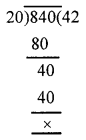The H.C.F. of 620, 720 and 840 is 20
∴ The required largest number = 20

Question 6.
Meenu purchases two bags of rice of weights 75 kg and 69 kg. Find the maximum value of weight which can measure the weight of the rice exact number of times.
Solution:
Weights of two bags = 75 kg, 69 kg⇒ 75 = 3 × 5 × 5
69 = 3 × 23
∴ H.C.F. of 75 and 69 = 3
⇒ The maximum value of weight which can measure the weight of the rice exact number of times is 3 kg.

Question 7.
Three tankers contain 403 litres, 434 litres and 465 litres of diesel respectively. Find the maximum capacity of a container that can measure the diesel of three containers exact number of times.
Solution:
Capacity of 3 tankers = 403 /, 434 /, 465 /
∴ Maximum capacity of a container, that can measure the diesel of three tankers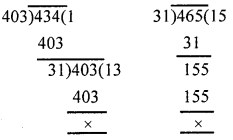= HCF of 403, 434, 465 = 31
∴Required measure = 31 litres